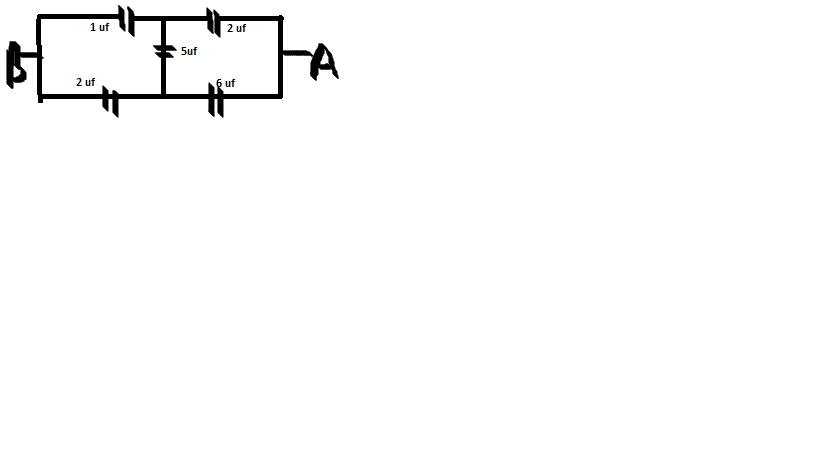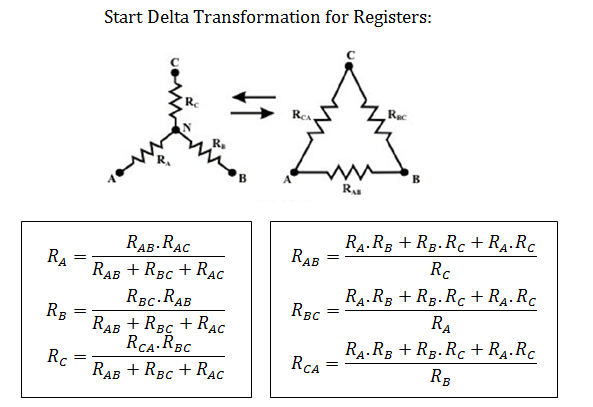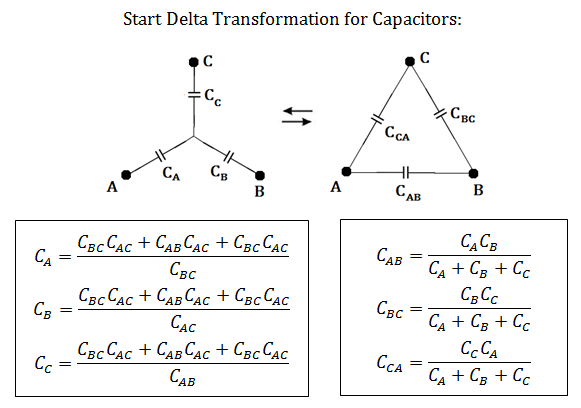# CapacitanceCalculate EQ capcitance . please solve

•I followed this method:
Let AB be connected to a 1 V battery.
then I distributed charge on all the capacitors, and wrote the KVL for (1) upper circuit, (2)lower circuit,
(3) and for 1,5,6 uF capacitors.

Now solving them I got the total charge to be 264/123=88/41=2.14 uC
which is the equivalent capacitance.

Akash Anand ·

Here is that formula you people were looking for.If you want to use the Y-Delta transformation for capacitors then just replace R by 1/C and you will get the corresponding result.••Sahil Jain ·

2.25 uf

••